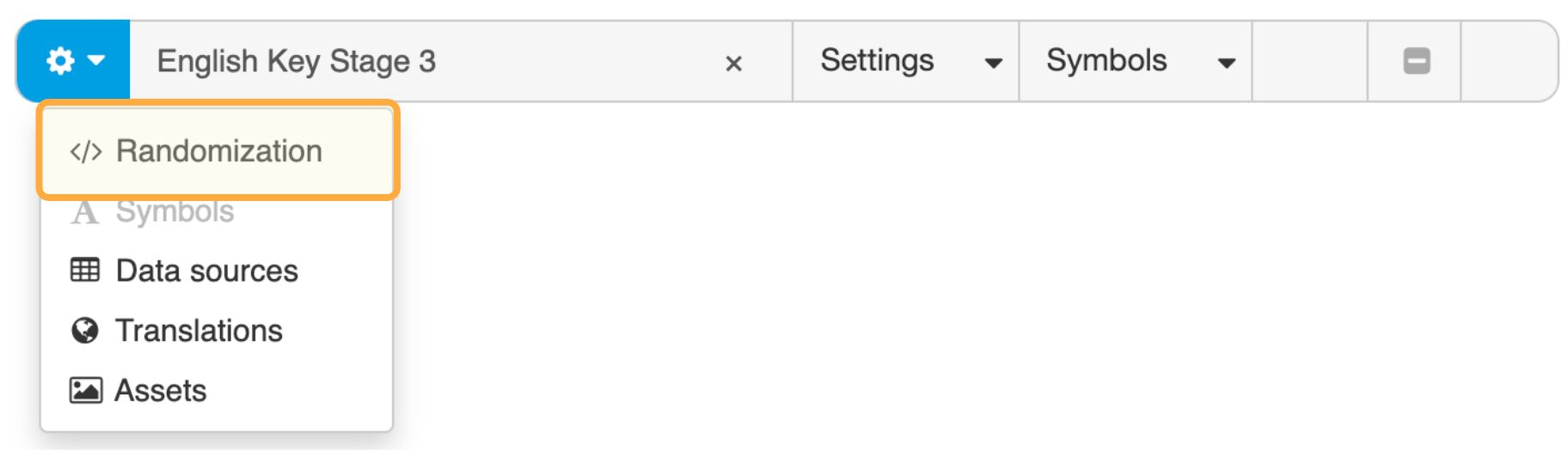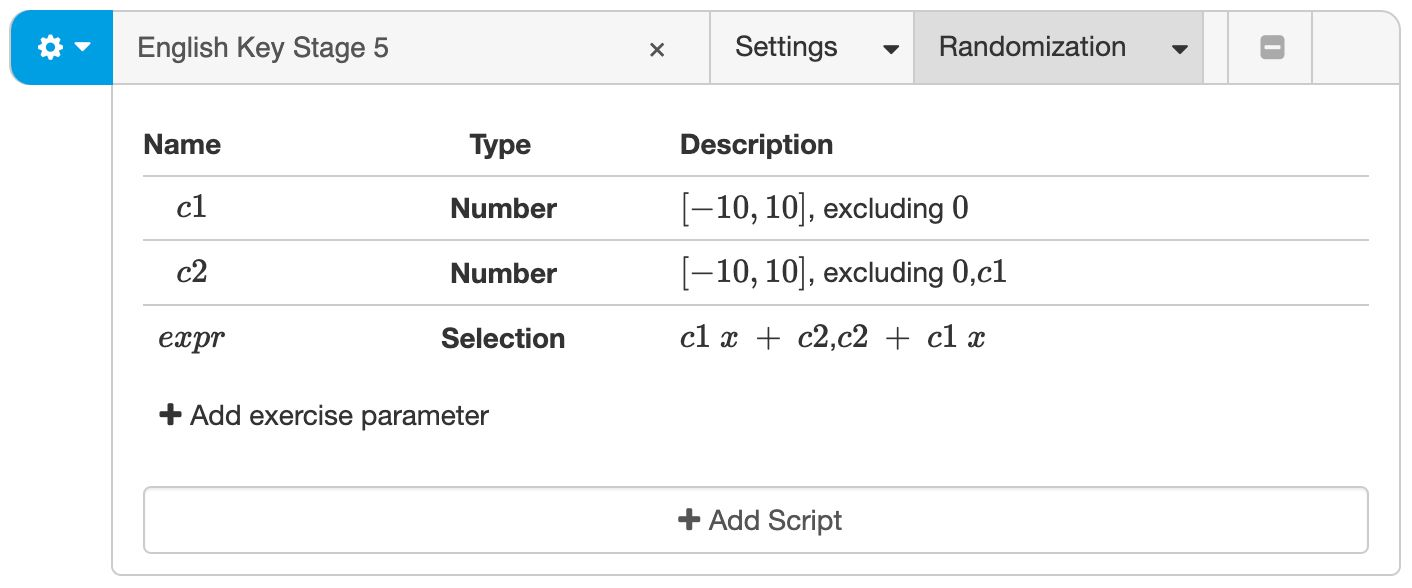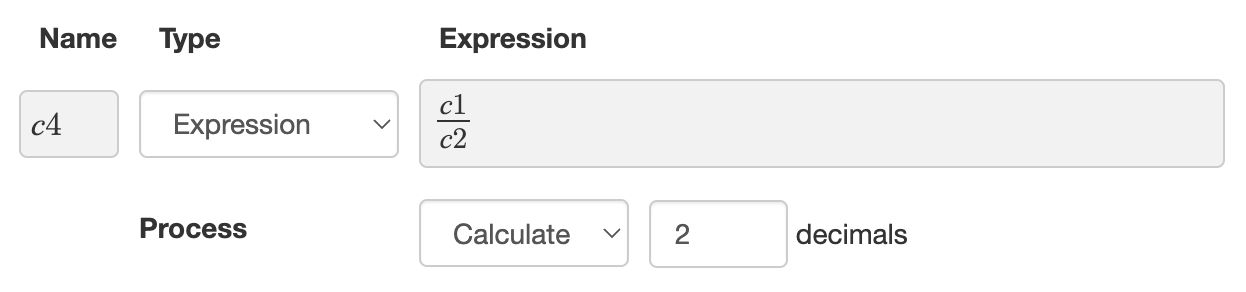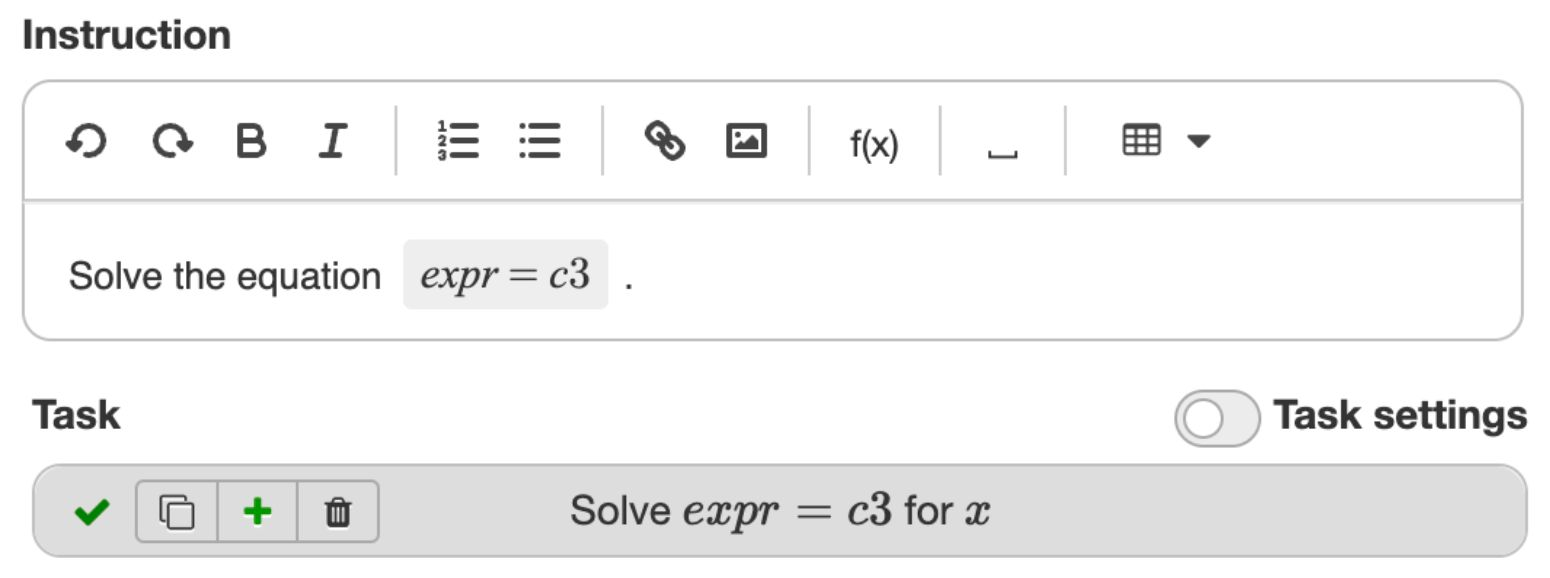# Randomizing Multistep

## Why random questions?

Algebrakit lets you create questions that are different every time. Random questions have several benefits, such as:

• Offer unlimited practice to master procedural fluency
• Create reusable assessment items.

Random questions offer the same functionalities as nonrandom ones, including substep evaluation and automated scaffolds.

Randomization is not limited to Multistep questions, but can be used in all question types. For example, you can use the Geometry & Graphs question type to display a random graph and use Multistep to let students write the corresponding function definition.

The live example below shows some exercises yo can try out. Use the Repeat button to generate new random instances.

Examples of random questions.

## Defining exercise parameters

You create random questions by defining exercise parameters and assign them random values. You define these exercise parameters in the Randomization panel in the top bar of the exercise. Add the panel with the settings dropdown button on the left if the panel is not already visible.Figure: Accessing the Randomization panel.Figure: Creating exercise parameters.

There are three types of exercise parameters:

• Number. The parameter will get a random integer number in the given range. You can add a list of numbers to exclude.
• Selection. The parameter will be a random expression from the given options. The options are not limited to numbers but can be any mathematical expression.
• Expression. The parameter will equal the given expression. Note that this option is not random.

Note that the definition of an exercise parameter can depend on any of the previous exercise parameters.Figure: Calculating and approximating an exercise parameter.

If the parameter is of type Selection or Expression, you may want to let Algebrakit simplify the expression. For example, if an exercise parameter has definition $c1 x + x2$, where $c1=1$ and $c2=0$, then the assigned value is $1x+0$. You probably want this to be simplified to $x$.

Another option is to let Algebrakit evaluate the parameter numerically. Consider for example an exercise parameter with definition $\frac{c1}{c2}$, where $c1=2$ and $c2=3$. You can let Algebrakit calculate and approximate the value.

## Using exercise parameters

You can use exercise parameters in any mathematical expression in your exercise. Every time a new question is started, Algebrakit will assign definitions to the exercise parameters and substitute the exercise parameters in the exercise.Figure: Using exercise parameters in a question.